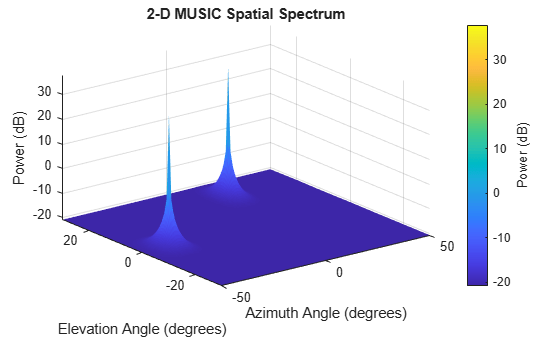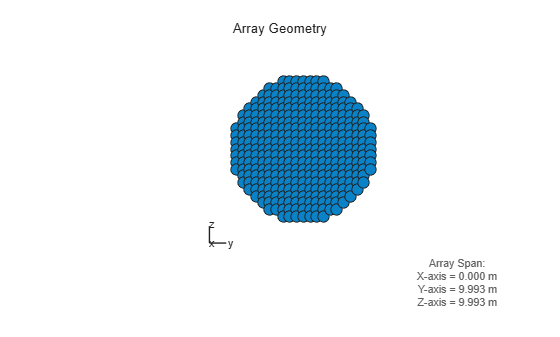# phased.MUSICEstimator2D

Estimate 2D direction of arrival using narrowband MUSIC algorithm

## Description

The `phased.MUSICEstimator2D` System object™ implements the narrowband multiple signal classification (MUSIC) algorithm for 2-D planar or 3-D arrays such as a uniform rectangular array (URA). MUSIC is a high-resolution direction-finding algorithm capable of resolving closely-spaced signal sources. The algorithm is based on the eigenspace decomposition of the sensor covariance matrix.

To estimate directions of arrival (DOA):

1. Define and set up a `phased.MUSICEstimator2D` System object. See Construction.

2. Call the `step` method to estimate the DOAs according to the properties of `phased.MUSICEstimator2D`.

Note

Instead of using the `step` method to perform the operation defined by the System object, you can call the object with arguments, as if it were a function. For example, `y = step(obj,x)` and `y = obj(x)` perform equivalent operations.

## Construction

`estimator = phased.MUSICEstimator2D` creates a MUSIC DOA estimator System object, `estimator`.

`estimator = phased.MUSICEstimator2D(Name,Value)` creates a System object, `estimator`, with each specified property Name set to the specified Value. You can specify additional name-value pair arguments in any order as (`Name1`,`Value1`,...,`NameN`,`ValueN`).

## Properties

expand all

Sensor array, specified as a Phased Array System Toolbox array System object.

Example: `phased.URA`

Signal propagation speed, specified as a real-valued positive scalar. Units are in meters per second. The default propagation speed is the value returned by `physconst('LightSpeed')`.

Example: `3e8`

Data Types: `single` | `double`

Operating frequency, specified as a positive scalar. Units are in Hz.

Example: `1e9`

Data Types: `single` | `double`

Enable forward-backward averaging, specified as `false` or `true`. Set this property to `true` to use forward-backward averaging to estimate the covariance matrix for sensor arrays with a conjugate symmetric array manifold.

Data Types: `logical`

Azimuth scan angles, specified as a or real-valued row vector. Angle units are in degrees. The angle values must lie between –180° and 180°, inclusive, and be in ascending order.

Example: `[-30:20]`

Data Types: `single` | `double`

Elevation scan angles, specified as a real-valued row vector. Angle units are in degrees. The angle values must lie between –90° and 90°, inclusive, and be in ascending order.

Example: `[-70:75]`

Data Types: `single` | `double`

Option to enable directions-of-arrival (DOA) output, specified as `false` or `true`. To obtain the DOA of signals, set this property to `true`. The DOAs are returned in the second output argument when the object is executed.

Data Types: `logical`

Source of the number of arriving signals, specified as `'Auto'` or `'Property'`.

• `'Auto'` — The System object estimates the number of arriving signals using the method specified in the `NumSignalsMethod` property.

• `'Property'` — Specify the number of arriving signals using the `NumSignals` property.

Data Types: `char`

Method used to estimate the number of arriving signals, specified as `'AIC'` or `'MDL'`.

• `'AIC'` — Akaike Information Criterion

• `'MDL'` — Minimum Description Length criterion

#### Dependencies

To enable this property, set `NumSignalsSource` to `'Auto'`.

Data Types: `char`

Number of arriving signals for DOA estimation, specified as a positive integer.

Example: `3`

#### Dependencies

To enable this property, set `NumSignalsSource` to `'Property'`.

Data Types: `single` | `double`

## Methods

 plotSpectrum Plot 2-D MUSIC spectrum reset Reset states of System object step Estimate direction of arrival using 2-D MUSIC
Common to All System Objects
`release`

Allow System object property value changes

## Examples

collapse all

Assume that two sinusoidal waves of frequencies 450 Hz and 600 Hz strike a URA from two different directions. Signals arrive from -37° azimuth, 0° elevation and 17° azimuth, 20° elevation. Use 2-D MUSIC to estimate the directions of arrival of the two signals. The array operating frequency is 150 MHz and the signal sampling frequency is 8 kHz.

```f1 = 450.0; f2 = 600.0; doa1 = [-37;0]; doa2 = [17;20]; fc = 150e6; c = physconst('LightSpeed'); lam = c/fc; fs = 8000;```

Create the URA with default isotropic elements. Set the frequency response range of the elements.

```array = phased.URA('Size',[11 11],'ElementSpacing',[lam/2 lam/2]); array.Element.FrequencyRange = [50.0e6 500.0e6];```

Create the two signals and add random noise.

```t = (0:1/fs:1).'; x1 = cos(2*pi*t*f1); x2 = cos(2*pi*t*f2); x = collectPlaneWave(array,[x1 x2],[doa1,doa2],fc); noise = 0.1*(randn(size(x))+1i*randn(size(x)));```

Create and execute the 2-D MUSIC estimator to find the directions of arrival.

```estimator = phased.MUSICEstimator2D('SensorArray',array,... 'OperatingFrequency',fc,... 'NumSignalsSource','Property',... 'DOAOutputPort',true,'NumSignals',2,... 'AzimuthScanAngles',-50:.5:50,... 'ElevationScanAngles',-30:.5:30); [~,doas] = estimator(x + noise)```
```doas = 2×2 -37 17 0 20 ```

The estimated DOAs exactly match the true DOAs.

Plot the 2-D spatial spectrum.

`plotSpectrum(estimator);`Assume that two sinusoidal waves of frequencies 1.6 kHz and 1.8 kHz strike a disk array from two different directions. The spacing between elements of the disk is 1/2 wavelength. Signals arrive from -31° azimuth, -11° elevation and 35° azimuth, 55° elevation. Use 2-D MUSIC to estimate the directions of arrival of the two signals. The array operating frequency is 300 MHz and the signal sampling frequency is 8 kHz.

Note: This example runs only in R2016b or later. If you are using an earlier release, replace each call to the function with the equivalent `step` syntax. For example, replace `myObject(x)` with `step(myObject,x)`.

```f1 = 1.6e3; f2 = 1.8e3; doa1 = [-31;-11]; doa2 = [35;55]; fc = 300e6; c = physconst('LightSpeed'); lam = c/fc; fs = 8.0e3;```

Create a conformal array with default isotropic elements. First, create a URA to get the element positions.

```uraarray = phased.URA('Size',[21 21],'ElementSpacing',[lam/2 lam/2]); pos = getElementPosition(uraarray);```

Extract a subset of these to form an inscribed disk.

```radius = 10.5*lam/2; pos(:,sum(pos.^2) > radius^2) = [];```

Then, create the conformal array using these positions.

```confarray = phased.ConformalArray('ElementPosition',pos); viewArray(confarray)```Set the frequency response range of the elements.

`confarray.Element.FrequencyRange = [50.0e6 600.0e6];`

Create the two signals and add random noise.

```t = (0:1/fs:1.5).'; x1 = cos(2*pi*t*f1); x2 = cos(2*pi*t*f2); x = collectPlaneWave(confarray,[x1 x2],[doa1,doa2],fc); noise = 0.1*(randn(size(x)) + 1i*randn(size(x)));```

Create and execute the 2-D MUSIC estimator to find the directions of arrival.

```estimator = phased.MUSICEstimator2D('SensorArray',confarray,... 'OperatingFrequency',fc,... 'NumSignalsSource','Property',... 'DOAOutputPort',true,'NumSignals',2,... 'AzimuthScanAngles',-60:.1:60,... 'ElevationScanAngles',-60:.1:60); [~,doas] = estimator(x + noise)```
```doas = 2×2 35 -31 55 -11 ```

The estimated DOAs exactly match the true DOAs.

Plot the 2-D spatial spectrum.

`plotSpectrum(estimator);`expand all

## References

 Van Trees, H. L., Optimum Array Processing. New York: Wiley-Interscience, 2002.

## Extended Capabilities

Introduced in R2016b